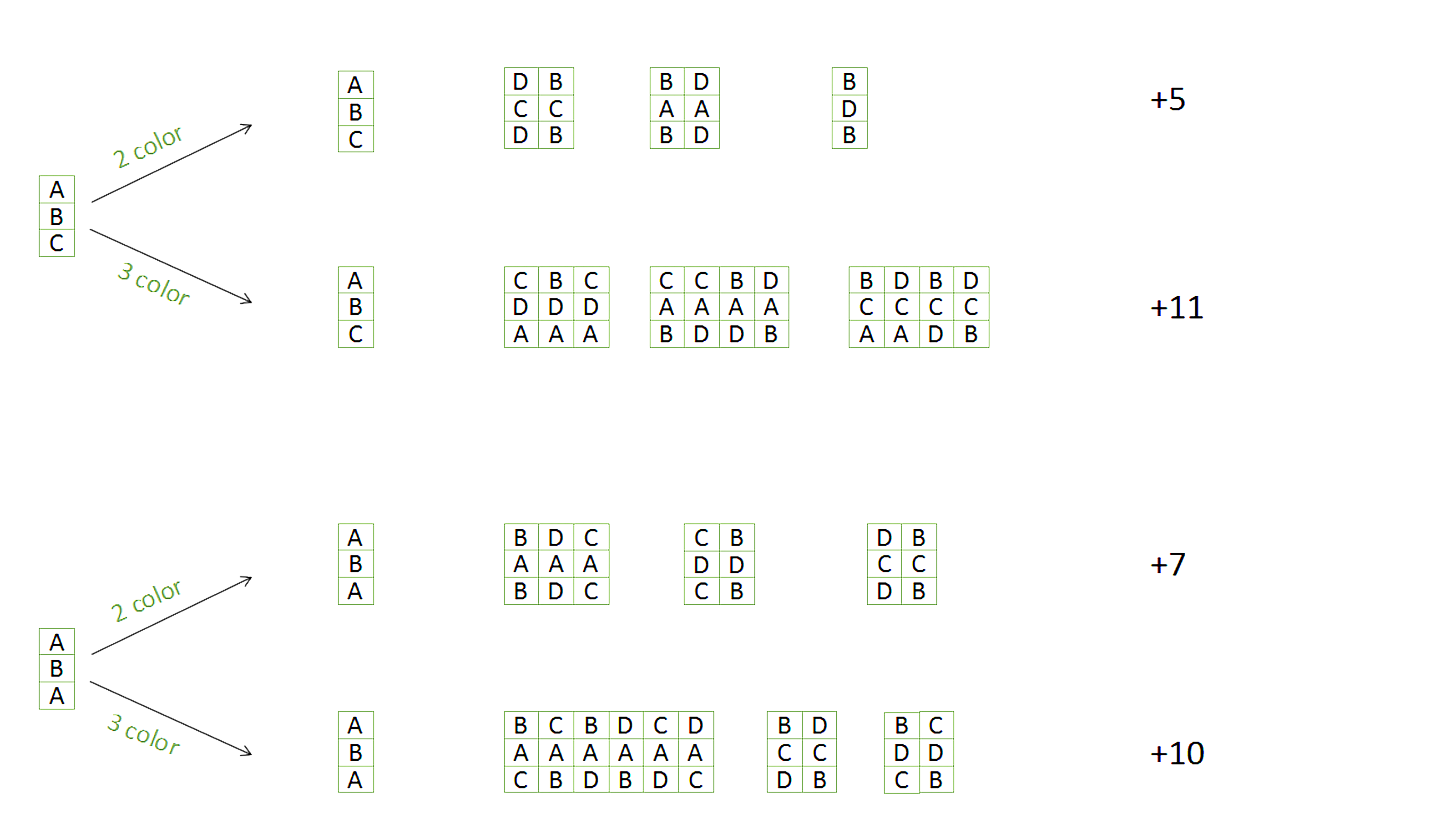# Ways to color a 3*N board using 4 colors

• Difficulty Level : Hard
• Last Updated : 28 Apr, 2021

Given a 3 X n board, find the number of ways to color it using at most 4 colors such that no two adjacent boxes have the same color. Diagonal neighbors are not treated as adjacent boxes.
Output the ways%1000000007 as the answer grows quickly.
Constraints:
1<= n < 100000
Examples :

```Input : 1
Output : 36
We can use either a combination of 3 colors
or 2 colors. Now, choosing 3 colors out of
4 isand arranging them

in 3! ways, similarly choosing 2 colors out

of 4 isand while arranging

we can only choose which of them could be at

centre, that would be 2 ways.

Answer =*3! +*2! = 36
Input : 2

Output : 588```

We are going to solve this using dynamic approach because when a new column is added to the board, the ways in which colors are going to be filled depends just upon the color pattern in the current column. We can only have a combination of two colors and three colors in a column. All possible new columns that can be generated is given in the image. Please consider A, B, C and D as 4 colors.All possible color combinations that can be generated from current column.

From now, we will refer 3 colors combination for a Nth column of the 3*N board as W(n) and two colors as Y(n).
We can see that each W can generate 5Y and 11W, and each Y can generate 7Y and 10W. We get two equation from here
We have two equations now,

```W(n+1) = 10*Y(n)+11*W(n);
Y(n+1) = 7*Y(n)+5*W(n);```

## C++

 `// C++ program to find number of ways``// to color a 3 x n grid using 4 colors``// such that no two adjacent have same``// color``#include ``using` `namespace` `std;` `int` `solve(``int` `A)``{``    ` `    ``// When we to fill single column``    ``long` `int` `color3 = 24;``    ``long` `int` `color2 = 12;``    ``long` `int` `temp = 0;``    ` `    ``for` `(``int` `i = 2; i <= A; i++)``    ``{``        ``temp = color3;``        ``color3 = (11 * color3 + 10 *``              ``color2 ) % 1000000007;``              ` `        ``color2 = ( 5 * temp + 7 *``              ``color2 ) % 1000000007;``    ``}``    ` `    ``long` `num = (color3 + color2)``                       ``% 1000000007;``                       ` `    ``return` `(``int``)num;``}` `// Driver code``int` `main()``{``    ``int` `num1 = 1;``    ``cout << solve(num1) << endl;` `    ``int` `num2 = 2;``    ``cout << solve(num2) << endl;``    ` `    ``int` `num3 = 500;``    ``cout << solve(num3) << endl;` `    ``int` `num4 = 10000;``    ``cout << solve(num4);``    ` `    ``return` `0;``}` `// This code is contributed by vt_m.`

## Java

 `// Java program to find number of ways to color``// a 3 x n grid using 4 colors such that no two``// adjacent have same color.``public` `class` `Solution {``    ``public` `static` `int` `solve(``int` `A) {``        ``long` `color3 = ``24``; ``// When we to fill single column``        ``long` `color2 = ``12``;``        ``long` `temp = ``0``;``        ``for` `(``int` `i = ``2``; i <= A; i++)       ``        ``{``            ``long` `temp = color3;``            ``color3 = (``11` `* color3 + ``10` `* color2 ) % ``1000000007``;``            ``color2 = ( ``5` `* temp + ``7` `* color2 ) % ``1000000007``;``        ``}``        ``long` `num = (color3 + color2) % ``1000000007``;``        ``return` `(``int``)num;``    ``}` `    ``// Driver code``    ``public` `static` `void` `main(String[] args)``    ``{``        ``int` `num1 = ``1``;``        ``System.out.println(solve(num1));` `        ``int` `num2 = ``2``;``        ``System.out.println(solve(num2));` `        ``int` `num3 = ``500``;``        ``System.out.println(solve(num3));` `        ``int` `num4 = ``10000``;``        ``System.out.println(solve(num4));``    ``}``}`

## Python3

 `# Python 3 program to find number of ways``# to color a 3 x n grid using 4 colors``# such that no two adjacent have same``# color` `def` `solve(A):``    ` `    ``# When we to fill single column``    ``color3 ``=` `24``    ``color2 ``=` `12``    ``temp ``=` `0``    ` `    ``for` `i ``in` `range``(``2``, A ``+` `1``, ``1``):``        ``temp ``=` `color3``        ``color3 ``=` `(``11` `*` `color3 ``+` `10` `*` `color2 ) ``%` `1000000007``            ` `        ``color2 ``=` `( ``5` `*` `temp ``+` `7` `*` `color2 ) ``%` `1000000007``    ` `    ``num ``=` `(color3 ``+` `color2) ``%` `1000000007``                        ` `    ``return` `num` `# Driver code``if` `__name__ ``=``=` `'__main__'``:``    ``num1 ``=` `1``    ``print``(solve(num1))``    ` `    ``num2 ``=` `2``    ``print``(solve(num2))``    ` `    ``num3 ``=` `500``    ``print``(solve(num3))` `    ``num4 ``=` `10000``    ``print``(solve(num4))``    ` `# This code is contributed by``# Shashank_Sharma`

## C#

 `// C# program to find number of ways``// to color a 3 x n grid using 4``// colors such that no two adjacent``// have same color.``using` `System;` `public` `class` `GFG {``    ` `    ``public` `static` `int` `solve(``int` `A)``    ``{``        ` `        ``// When we to fill single column``        ``long` `color3 = 24;``        ``long` `color2 = 12;``        ``long` `temp = 0;``        ` `        ``for` `(``int` `i = 2; i <= A; i++)``        ``{``            ``temp = color3;``            ``color3 = (11 * color3 + 10``                 ``* color2 ) % 1000000007;``                 ` `            ``color2 = ( 5 * temp + 7``                 ``* color2 ) % 1000000007;``        ``}``        ``long` `num = (color3 + color2)``                            ``% 1000000007;``        ``return` `(``int``)num;``    ``}` `    ``// Driver code``    ``public` `static` `void` `Main()``    ``{``        ``int` `num1 = 1;``        ``Console.WriteLine(solve(num1));` `        ``int` `num2 = 2;``        ``Console.WriteLine(solve(num2));` `        ``int` `num3 = 500;``        ``Console.WriteLine(solve(num3));` `        ``int` `num4 = 10000;``        ``Console.WriteLine(solve(num4));``    ``}``}` `// This code is contributed by vt_m.`

## PHP

 ``

## Javascript

 ``

Output :

```36
588
178599516
540460643```

This article is contributed by Panshul Garg. If you like GeeksforGeeks and would like to contribute, you can also write an article using contribute.geeksforgeeks.org or mail your article to review-team@geeksforgeeks.org. See your article appearing on the GeeksforGeeks main page and help other Geeks.